cancel
Showing results for
Did you mean:
cancel
Showing results for
Did you mean:
Developer forum

## Can I skip the value from the label to mark it?Contributor

Hi

I'm making a label for Finishing materials.

there is a problem to solve that I want to skip the value named AIR but i don't know how to skip it.For example, in this picture Even if the slab contains 4 materials, I don't want to label the material named AIR.

this is the scripts i used.

``````for i = 1 to numText

if (fra(MATthk[n]*1000) > -eps and fra(MATthk[n]*1000) < eps) then
thk = str("%.0mm", MATthk[n])
else
thk = str("%.1mm", MATthk[n])
endif

autotxt[i] = "T" + thk + " " + MATname[n]
autoTHK[i] = MATthk[n]``````

I don't see how to exclude the value MATname[n] = "AIR" in the middle of this root.

Even if i force it to make it by removing the value, it was only marked as zero, but it was not completely removed.

I want only three expressions: AA, BB, and CC on my label.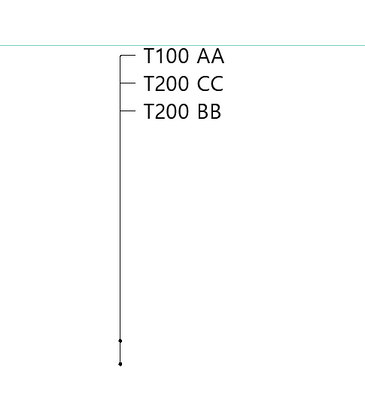Like this picture.

thank you.

++

I tried add some loop on script as someone adviced me before

but it still put "air" or "0" on the screen.

I want to SKIP the "air" line.

``````for i = 1 to numText
if MATname[i] = "공기 공간" then
autotxt[i] =autotxt
else
if (fra(MATthk[n]*1000) > -eps and fra(MATthk[n]*1000) < eps) then
thk = str("%.0mm", MATthk[n])
else
thk = str("%.1mm", MATthk[n])
endif
autotxt[i] = "T" + thk + " " + MATname[n]
autoTHK[i] = MATthk[n]
if mirrorText = 0 then
n = n+1
else
n = n-1
endif
endif

next i``````
5 REPLIES 5Contributor

Hi Soo,

Can you show how you have generated the texts? I am thinking that you should control this where you generate text.

`if MATname[i] = "공기 공간" thenelse!!GENERATE TEXT!!endif`

You could also try utilising ID field of building material. For instance the ID of building materials you want to hide could be set to "-" and in the script you can request building material ID and set it to do nothing if ID is "-".

`n = REQUEST{2} ("Building_Material_info",n = REQUEST{2} ("Building_Material_info", name_or_index, "gs_bmat_id", MY_MATERIAL_ID)`Cheers,

James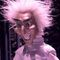It looks like you need to convert your data to a string and then exclude it with an if statement should the string not matchContributor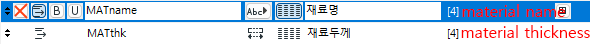this is how i put the material name and material thickness.

``````if MATname = "AIR"

then

else
for i = 1 to numText
if (fra(MATthk[n]*1000) > -eps and fra(MATthk[n]*1000) < eps) then
thk = str("%.0mm", MATthk[n])
else
thk = str("%.1mm", MATthk[n])
endif
autotxt[i] = "T" + thk + " " + MATname[n]
autoTHK[i] = MATthk[n]
if mirrorText = 0 then
n = n+1
else
n = n-1
endif
endif

if i = userTextPos1 then
autotxt[i] = userText1
autoTHK[i] = 0
endif
if i <> userTextPos1 then
if (fra(MATthk[n]*1000) > -eps and fra(MATthk[n]*1000) < eps) then
thk = str("%.0mm", MATthk[n])
else
thk = str("%.1mm", MATthk[n])
endif
autotxt[i] = "T" + thk + " " + MATname[n]
autoTHK[i] = MATthk[n]
if mirrorText = 0 then
n = n+1
else
n = n-1
endif
endif
endif
if i = userTextPos1 then
autotxt[i] = userText1
autoTHK[i] = 0
endif
if i = userTextPos2 then
autotxt[i] = userText2
autoTHK[i] = 0
endif
if i <> userTextPos1 and i <> userTextPos2 then
if (fra(MATthk[n]*1000) > -eps and fra(MATthk[n]*1000) < eps) then
thk = str("%.0mm", MATthk[n])
else
thk = str("%.1mm", MATthk[n])
endif
autotxt[i] = "T" + thk + " " + MATname[n]
autoTHK[i] = MATthk[n]
if mirrorText = 0 then
n = n+1
else
n = n-1
endif
endif
endif
if i = userTextPos1 then
autotxt[i] = userText1
autoTHK[i] = 0
endif
if i = userTextPos2 then
autotxt[i] = userText2
autoTHK[i] = 0
endif
if i = userTextPos3 then
autotxt[i] = userText3
autoTHK[i] = 0
endif
if i <> userTextPos1 and i <> userTextPos2 and i <> userTextPos3 then
if (fra(MATthk[n]*1000) > -eps and fra(MATthk[n]*1000) < eps) then
thk = str("%.0mm", MATthk[n])
else
thk = str("%.1mm", MATthk[n])
endif
autotxt[i] = "T" + thk + " " + MATname[n]
autoTHK[i] = MATthk[n]
if mirrorText = 0 then
n = n+1
else
n = n-1
endif
endif
endif
if i = userTextPos1 then
autotxt[i] = userText1
autoTHK[i] = 0
endif
if i = userTextPos2 then
autotxt[i] = userText2
autoTHK[i] = 0
endif
if i = userTextPos3 then
autotxt[i] = userText3
autoTHK[i] = 0
endif
if i = userTextPos4 then
autotxt[i] = userText4
autoTHK[i] = 0
endif
if i <> userTextPos1 and i <> userTextPos2 and i <> userTextPos3 and i <> userTextPos4 then
if (fra(MATthk[n]*1000) > -eps and fra(MATthk[n]*1000) < eps) then
thk = str("%.0mm", MATthk[n])
else
thk = str("%.1mm", MATthk[n])
endif
autotxt[i] = "T" + thk + " " + MATname[n]
autoTHK[i] = MATthk[n]
if mirrorText = 0 then
n = n+1
else
n = n-1
endif
endif
endif
if i = userTextPos1 then
autotxt[i] = userText1
autoTHK[i] = 0
endif
if i = userTextPos2 then
autotxt[i] = userText2
autoTHK[i] = 0
endif
if i = userTextPos3 then
autotxt[i] = userText3
autoTHK[i] = 0
endif
if i = userTextPos4 then
autotxt[i] = userText4
autoTHK[i] = 0
endif
if i = userTextPos5 then
autotxt[i] = userText5
autoTHK[i] = 0
endif
if i <> userTextPos1 and i <> userTextPos2 and i <> userTextPos3 and i <> userTextPos4 and i <> userTextPos4 then
if (fra(MATthk[n]*1000) > -eps and fra(MATthk[n]*1000) < eps) then
thk = str("%.0mm", MATthk[n])
else
thk = str("%.1mm", MATthk[n])
endif
autotxt[i] = "T" + thk + " " + MATname[n]
autoTHK[i] = MATthk[n]
if mirrorText = 0 then
n = n+1
else
n = n-1
endif
endif
endif
next i
endif``````i did that you wrote it before.

and now it shows like this.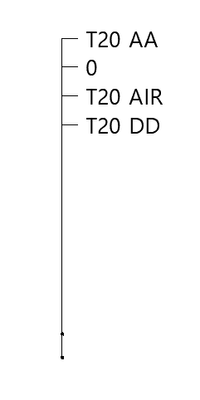if you know how to pass the "AIR" away,Contributor

Hello I want to remove a specific value from an array.

For example, if the Label object can show Material Thickness and Material name togeter.

I removed only the "air" layer to don't display it on screen.

I asked before twice in this board.

but the info i got last time, it just shows the value as 0, but I couldn't get rid of it.->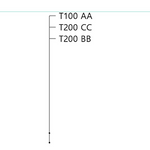i wanna make this label which can remove "AIR" row automatically.

I attach my file below.

plz let me know how to solve this problemGraphisoft

Hi,

GDL doesn't have the equivalent of break/continue in other languages.

It would be simpler to add a condition where you are displaying the data with TEXT2.

Or you need to be creative with goto:

``````output = 1
for input = ...
if MATName[input] = "AIR" then goto "nextMAT" ! skip input
! process input
autotext[output] = ...
...
output = output + 1
"nextMAT":
next input

``````

Péter Baksa
Software Engineer, Library as a Platform
Graphisoft SE, BudapestLatest solutions

Most liked posts